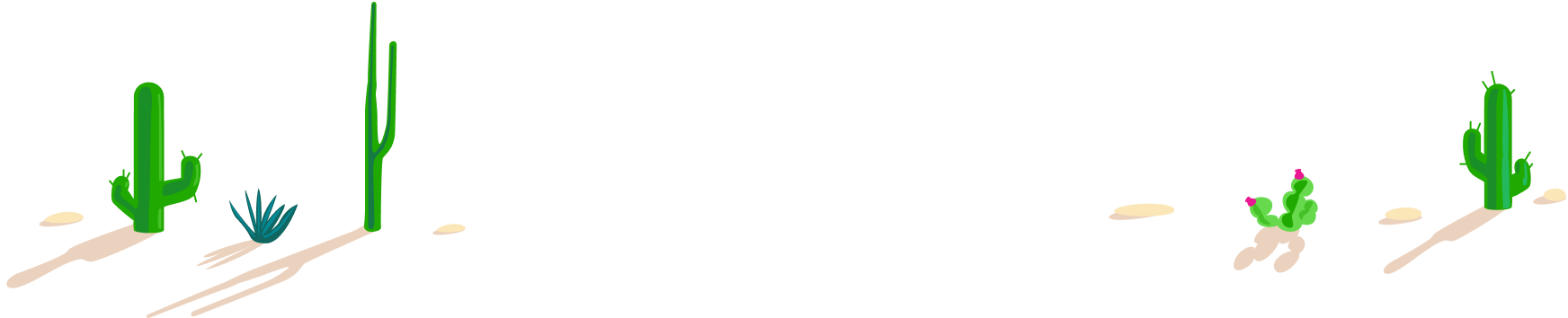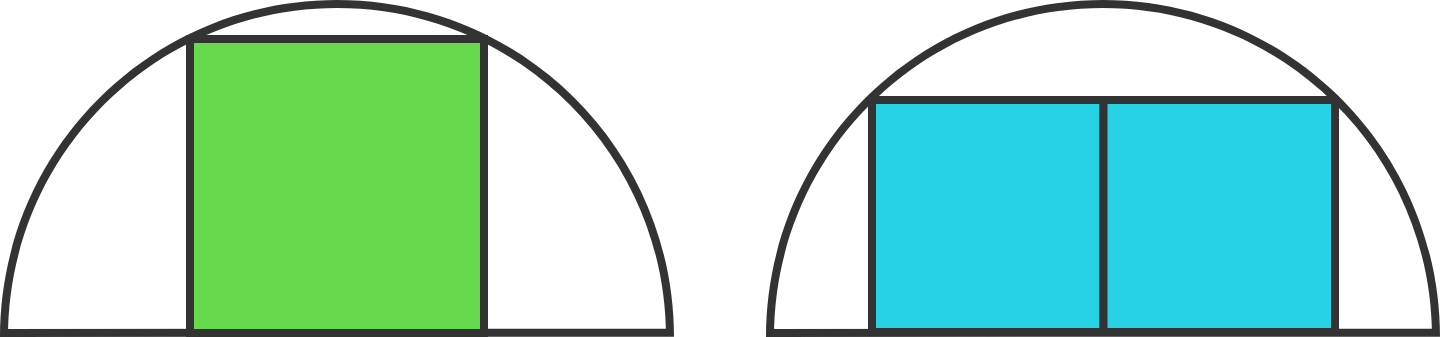# 100 Day Summer Challenge

## 100 problems in 100 days. #100problems

Day 81# 81 of 100: Ratio Riddle

Geometry Level 2

Two identical semicircles circumscribe one square and a rectangle composed of two squares, respectively.What is the ratio of the green area to the blue area?Hint: Look for ways to apply the Pythagorean theorem.

BONUS: Following the same pattern we've introduced for 1 and 2 squares, for what number of squares is the area of the squares the largest?